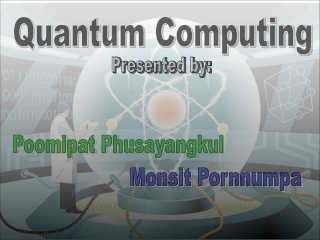DownloadDownload PresentationQuantum Computing

# Quantum Computing

Télécharger la présentation## Quantum Computing

- - - - - - - - - - - - - - - - - - - - - - - - - - - E N D - - - - - - - - - - - - - - - - - - - - - - - - - - -
##### Presentation Transcript

1. Quantum Computing Presented by: Poomipat Phusayangkul Monsit Pornnumpa

2. Richard P. Feynman Charles H. Bennett David Deutsch History of QuanTum Computer • The idea of a computational by physicists and computer scientists like: Charles H. Bennett Paul A. Benioff David Deutsch Richard P. Feynman 1982:Feynman explained how such quantum computer would be able to act as a simulator for quantum physics. 1985:Deutsch realized that Feynman's assertion could eventually lead to a general purpose quantum computer and published a crucial theoretical paper. 1994: Unfortunately, all that could be found were a few rather contrived mathematical problems.

3. Device for computation that makes direct use of • superpositionandentanglement, to perform • operations on data. • In a quantum computer, the amount of data is • measured byqubits. • Quantum properties of particles can be used to • represent and structure data. WHAT is QUANTUM COMPUTER?

4. Coherent Superposition • A qubit can exist in states corresponding to a blend or superposition of these classical • states. • A qubit can exist simultaneously as both 0 and 1. Experiment of Coherent Superposition:

5. Entanglement • A quantum mechanical phenomenon in which of two or more objects reference to each other. • It is possible to prepare two particles in a single quantum state such when one is spin-up, another one will always spin-down and vice versa. As a result, measurements performed on one system seem to be instantaneously influencing other systems entangled with it. Closely concerned with the emerging technologies of quantum computing, cryptography, and teleportation.

6. Classical VS Quantum • Consider first a classical computer that operates on a 3 bit • register. • Qubits can be in a superposition of all the classically allowed states. For an n qubit quantum register, recording the state of the register requires 2n complex numbers. Number of classical states encoded in a quantum register grows exponentially with the number of qubits.

7. Quantum computer could solve Integer factorization problem easily. • Most of the popular public key ciphers could be much more quickly broken, including forms of RSA, ElGamaland Diffie-Hellman. The time for a quantum computer to solve a problem will, reducing from years to seconds. Quantum chip ! QUANTUM POWER ! • Consider a problem that has these four properties: • The only way to solve it is to guess answers repeatedly and check them. • There are n possible answers to check. • Every possible answer takes the same amount of time to check. • There are no clues about which answers might be better: generating possibilities randomly is just as good as checking them in some special order.

8. an ion-trap the core of a quantum computer Obstacle in building Quantum Computer • The field of quantum information processing has made numerous promising advancements since its conception, However, a few potentially large obstacles still remain that prevent us from just building one. Below are the list of requirements for a practical quantum computer: • scalable physically to increase the number of • qubits • qubits can be initialized to arbitrary values • quantum gates faster than decoherence time • Turing-complete gate set • qubits can be read easily What errors need correction? To date, the designs of quantum computer involved ion traps, cavity quantum electrodynamics (QED), and NMR.

9. Reference http://en.wikipedia.org/wiki/Quantum_computer http://www.cs.caltech.edu/~westside/quantum-intro.html In Thai http://www.kitty.in.th/index.php?room=article&id=80 THE END